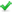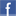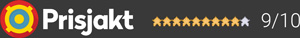#### Inga produkter tillagda!

Lägg en produkt i varukorgen så ser det lite roligare ut här ;)

#### Varukorg

0 kr# CayinCS-88A

24 990 kr/st
Välj utförande
24 990 kr24 990 krFri frakt!

CS-88A

Hand-made construction with the finest point-to-point wiring.

Two EI output transformers with wide bandwidth are applied.

Specially designed toroid transformer for power supply.

Two 6SL7 and two 6SN7 tubes are used in the preamp stage.

Four KT88/6550EH tubes are used for AB1 push-pull amplification.

Triode and Ultralinear selection.

1 x Phono ingång (MM)

External user Bias Adjustment is accessible with the BIAS meter for monitoring on the top of the amplifier.

External Tube Selection Switch between EL34 and KT88 on the rear panel for easy tube replacement.

Equipped with remote control.

Can be used as a Power amplifier.

Soft start function (about 30 seconds).

CS-88A can memorize last operation of the unit once turned off.

High-quality Japanese ALPS motor potentiometer.

Thickly silver-plated signal cable has been used.

Equipped with easily-installed tube cover for protection.Dela på Facebook

## Specifikationer

Förstärkare
ModellCS-88A
Effekt27+27 (Triode) 48+48 (Ultra-linear) Watt
Frekvensomfång10-42 (-3 dB) Hz
Signal/Brusförhållande93 dB
Strömförbrukning standby/drift<280 Watt
Dimensioner (B x H x D420×195×382 mm
Vikt28 kg

#### Frågor? Ring 031-241600eller maila //<![CDATA[ var l=new Array(); l = '>'; l = 'a'; l = '/'; l = '<'; l = '|109'; l = '|111'; l = '|99'; l = '|46'; l = '|110'; l = '|114'; l = '|97'; l = '|108'; l = '|108'; l = '|97'; l = '|107'; l = '|100'; l = '|117'; l = '|106'; l = '|108'; l = '|64'; l = '|111'; l = '|102'; l = '|110'; l = '|105'; l = '>'; l = '"'; l = '|109'; l = '|111'; l = '|99'; l = '|46'; l = '|110'; l = '|114'; l = '|97'; l = '|108'; l = '|108'; l = '|97'; l = '|107'; l = '|100'; l = '|117'; l = '|106'; l = '|108'; l = '|64'; l = '|111'; l = '|102'; l = '|110'; l = '|105'; l = ':'; l = 'o'; l = 't'; l = 'l'; l = 'i'; l = 'a'; l = 'm'; l = '"'; l = '='; l = 'f'; l = 'e'; l = 'r'; l = 'h'; l = ' '; l = 'a'; l = '<'; for (var i = l.length-1; i >= 0; i=i-1) { if (l[i].substring(0, 1) === '|') document.write("&#"+unescape(l[i].substring(1))+";"); else document.write(unescape(l[i])); } //]]>